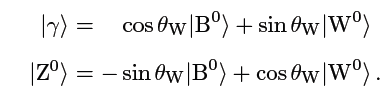# Small questions about weinberg angle !

goinginsane
Hello !

My books defines the photon and Z0 boson as:I have 3 questions

Are B0 and W0 orthogonal or the photon and Z0?
How do you derive that tg(t) = g'/g (with g' corresponding to B0, g to W0)
How do you derive that e = sin(t) ?

Also W0 couples with g (same as the "normal" W+ and W-) and B0 couples with g'.
My first question is (rather dumb one but its getting late and can't think clearly anymore haha):
Are W0 and B0 orthogonal or the foton and Z0 eigenfunctions?

Second question is: My book said ==> the photon only interacts with electrical charge
I said "yes, that's very true mister book! You are finally starting to understand it !
Then he said : well, like I was saying " the photon only interacts with electrical charge, this implies that :
tg(t) = g'/g

I said " Mister book, I am sorry if I have offended you but now you are just making stuff up ! "
He answered " HAHA ! I guess YOU are the one that doesn't understand it"
Anyways we kibbeled for a long time more...
How can you derive that ?I shut my book (up) and i tried to understand it like this :

for example define weak interaction operator A

<f|A|f> = 0 (cause doesn't interact) = cos(t) <B0|A|B0> + sin(t) <Z0|A|Z0> (With assuming B0 and Z0 were orthogonal.)
so you get 0 = g' cos(t) + g sin(t)
But then ofcourse i get a minus. I had the feeling i was doing a good job.

So i opened the book again and showed him my progress. But alas, he laughed again.
Cause under that formula there was e = sin(t) g and now I am really confused !
I threw the sadistic book in the closest as punishment. And I fear I won't be able to open it again without understanding it first.

Kind regards,

an idiot :)

Last edited:

goinginsane, I'm thinking this may be an assignment, is it? So I'll just sketch the idea. Write out the neutral current interaction, Lint ~ g JμW0μ + g' JμY Bμ. (I'm deliberately leaving out some factors.) Use the weak-angle rotation to express W0μ and Bμ in terms of Aμ and Zμ: Lint = (...)Aμ + (...)Zμ. The coefficient in front of Aμ must be the electric current, e(Jμ + JμY). (Factors omitted.) Equating this to the expression you just derived should give the relationships between e, g and g'.

goinginsane
goinginsane, I'm thinking this may be an assignment, is it? So I'll just sketch the idea. Write out the neutral current interaction, Lint ~ g JμW0μ + g' JμY Bμ. (I'm deliberately leaving out some factors.) Use the weak-angle rotation to express W0μ and Bμ in terms of Aμ and Zμ: Lint = (...)Aμ + (...)Zμ. The coefficient in front of Aμ must be the electric current, e(Jμ + JμY). (Factors omitted.) Equating this to the expression you just derived should give the relationships between e, g and g'.

No it is not, we don't get assigments just an exam :)
I could just read it and assume its correct. but I always try to derive all equations by myself when I am learning something.
thanks for the help anyways

aha, well this is just introduction to particle physics so I might be asking questions i can't understand yet. sorry :P